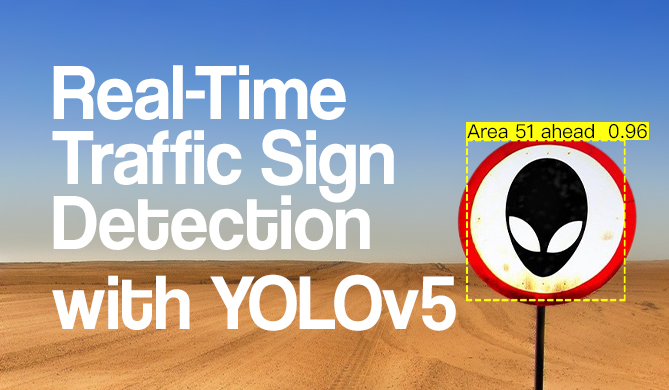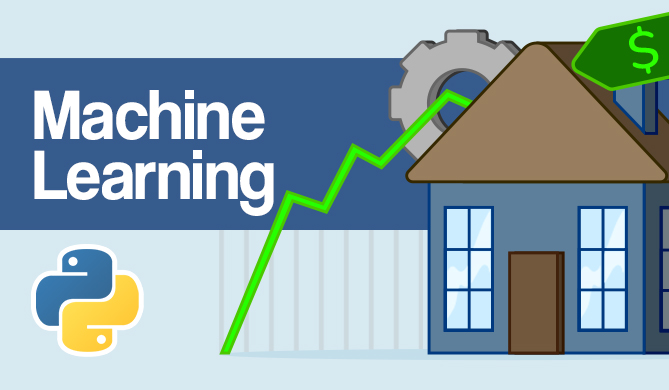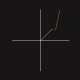Agglomerative Hierarchical Clustering in Python with Scikit-Learn

# Agglomerative Hierarchical Clustering in Python with Scikit-LearnAgglomerative Hierarchical Clustering is an unsupervised learning algorithm that links data points based on distance to form a cluster, and then links those already clustered points into another cluster, creating a structure of clusters with subclusters.

It is easily implemented using Scikit-Learn which already has single, average, complete and ward linking methods available.

If you'd like to read an in-depth guide to Hierarchical Clustering, read our Hierarchical Clustering with Python and Scikit-Learn"!

To visualize the hierarchical structure of clusters, you can load the Palmer Penguins dataset, choose the columns that will be clustered, and use Scipy to plot a Dendrogram of the subclusters.Let's import the libraries and load the Penguins dataset, trimming it to the chosen columns and dropping rows with missing data (there were only 2):

import pandas as pd
import seaborn as sns
import matplotlib.pyplot as plt
from sklearn.cluster import AgglomerativeClustering
from scipy.cluster import hierarchy

print(df.shape) # (344, 9)
df = df[['bill_length_mm', 'flipper_length_mm']]
df = df.dropna(axis=0)


We can use Scipy's hierarchy.linkage() to form clusters and plot them with hierarchy.dendrogram():

clusters = hierarchy.linkage(df, method="ward")

plt.figure(figsize=(8, 6))
dendrogram = hierarchy.dendrogram(clusters)
# Plotting a horizontal line based on the first biggest distance between clusters
plt.axhline(150, color='red', linestyle='--');
# Plotting a horizontal line based on the second biggest distance between clusters
plt.axhline(100, color='crimson');


This example shows how the Dendrogram is only a reference when used to choose the number of clusters. We already know that we have 3 types of penguins in the dataset, but if we were to determine their number by the Dendrogram, 2 would be our first option, and 3 would be our second option.

Now, let's perform Agglomerative Clustering with Scikit-Learn to find cluster labels for the three types of penguins:

## Get free courses, guided projects, and more

clustering_model = AgglomerativeClustering(n_clusters=3, linkage="ward")
clustering_model.fit(df)
labels = clustering_model.labels_


And plot the data before and after Agglomerative Clustering with 3 clusters:

fig, axes = plt.subplots(nrows=1, ncols=2, figsize=(15,5))
sns.scatterplot(ax=axes, data=df, x='bill_length_mm', y='flipper_length_mm').set_title('Without cliustering')
sns.scatterplot(ax=axes, data=df, x='bill_length_mm', y='flipper_length_mm', hue=clustering_model.labels_).set_title('With clustering');


When using Agglomerative Clustering, you don't need to pre-determine the number of clusters. As we have seen in the Dendrogram, if we don't determine how many clusters we aim to have, the algorithm will usually divide points into 2 clusters.

Let's try Agglomerative Clustering without specifying the number of clusters, and plot the data without Agglomerative Clustering, with 3 clusters and with no pre defined clusters:

clustering_model_no_clusters = AgglomerativeClustering(linkage="ward")
clustering_model_no_clusters.fit(df)
labels_no_clusters = clustering_model_no_clusters.labels_


And finally, let's plot the data without Agglomerative Clustering, with 3 clusters and with no pre defined clusters:

fig, axes = plt.subplots(nrows=1, ncols=3, figsize=(15,5))
sns.scatterplot(ax=axes, data=df, x='bill_length_mm', y='flipper_length_mm').set_title('Without cliustering')
sns.scatterplot(ax=axes, data=df, x='bill_length_mm', y='flipper_length_mm', hue=clustering.labels_).set_title('With 3 clusters')
sns.scatterplot(ax=axes, data=df, x='bill_length_mm', y='flipper_length_mm', hue=clustering_model_no_clusters.labels_).set_title('Without choosing number of clusters');

Last Updated: July 2nd, 2022Project

### Real-Time Road Sign Detection with YOLOv5

# python# machine learning# computer vision# pytorch

If you drive - there's a chance you enjoy cruising down the road. A responsible driver pays attention to the road signs, and adjusts their...David Landup
DetailsProject

### Hands-On House Price Prediction - Machine Learning in Python

# python# machine learning# scikit-learn# tensorflow

If you've gone through the experience of moving to a new house or apartment - you probably remember the stressful experience of choosing a property,...Details